• Archimedean screws and systems for raising water, from Leonardo da Vinci, Codex Atlanticus, f. 26 v. Milan, Biblioteca Ambrosiana.

Home | Didactics | Classes | Hydraulics (M.D. 270) - First Level Degree in Civil and Environmental Engineering (Taranto)Didactics

Classes

Hydraulics (M.D. 270) - First Level Degree in Civil and Environmental Engineering (Taranto)964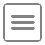• The students of Hydraulics of the Academic Year 2015-2016.

Scientific Sector
ICAR/01 Hydraulics

Number of University Credits
12

Educational Goals
The course deals with fundamental issues in the Degree Class of Civil and Environmental Engineering, of which is an essential and distinctive educational activity. The teaching of hydraulics provides engineering students with the basics of the fluid mechanics and tools of the applied hydraulics. The program is divided into four parts. In the first part the basic principles of fluid mechanics and hydraulics laws governing the fluid motion are provided.
In the second part the typical applications of steady and unsteady flows in pipes, local and net head loss and the unsteady flows in are presented. In the third part the course provides the students with the basics of the steady flows in open channels.
In the third part the open-channel topics are presented and typical problems are solved, with the analysis of the steady flow, the gradually varied flow profiles and the unsteady flow.
In the fourth part the course aims to provide the fundamentals of physical hydraulic modeling, with the principles of the dimensional analysis, the geometric, kinematic and dynamic similarity. In addition, students are given the skills necessary for understanding the fluid dynamics through laboratory training.
In addition, students are given the skills necessary for understanding the fluid dynamics through laboratory training.

Summary of the program
BASICS OF HYDRAULICS (2.5 credits)
• Preliminary remarks. Physical properties of fluids. Hydrostatic.
• Equilibrium of a fluid element and integral relation for a control volume.
• Hydrostatic forces on plane and curved surfaces.
• Kinematics of fluids. Conservation of mass with differential and integral relations.
• Dynamics of frictionless and Newtonian flows. Turbulent flows. Differential and integral equations of linear momentum. Forces in dynamic conditions.
VISCOUS FLOW IN DUCTS (5 credits)
• Velocity and discharge measurements.
• Steady flows in long circular ducts.
• Total and local head losses.
• Pipe network: typical design and check problems.

OPEN CHANNEL FLOWS (3.5 credit)
• Specific energy.
• Steady flows in open channels.
• Uniform flows and design of channels.
PHYSICAL HYDRAULIC MODELING (1 credit)
• Basics. Dimensional analysis and Pi theorem.
• Geometric, kinematic and dynamic similarity.
• Principles of the physical model construction.
Preparatory exams
Lab training requirements
The activities of lab training are required, since they are fundamental also the theoretical part of the course.

Structure in different typological educational activities
 Educational activities Credits Didactics (hours) Studying (hours) Theoretical classes 9 72 153 Exercises and seminars 2,5 40 22,5 Laboratory training 0,5 12 0,5 Total 12 124 176

Final examination
The final exam of Hydraulics is composed of:
1) Three written tests on each of the following subjects: a) hydrostatic forces, b) dynamic forces and c) design of multiple-pipe systems and channels.
2) Oral test on the theoretical part of the course.
3) Lab training (the lab experiments are carried out only during the course at the Hydraulic Laboratory of the faculty).
During the classes three written tests (exemptions from the examination written tests) will be proposed to the students who attend the lessons (always on each of the following subjects: a) hydrostatic forces, b) dynamic forces and c) Design of multiple-pipe systems and channels). The students that pass these three tests will be successively examined only orally (but only at the exams of January and February) on the theoretical  part of the course. In case of fail of one or more of the exemption tests (also for absence), the students will be examined also with the relating written tests.
The students are kindly reminded that if they take the written test they must take the oral test only on the aforementioned date.
During the final examination the students could also present a report describing the laboratory experiments.

Detailed program of the course
Basics of fluids. Difference of characteristics between solids, liquids and gases. Shear stresses in fluids. Proof of the theorem of Cauchy tetrahedron. Physical properties of fluids: density and specific gravity.
Corollary of the theorem of Cauchy tetrahedron; hydrostatic case: the principle of Pascal. Compressibility of liquids. Compressibility of gas. Index number of Mach. Introduction to surface tension: definition; practical cases. Contact angle effects. Liquids wetting and notwetting the solid. Proof of the equation of Laplace.
Proof of the law of Jurin-Borelli: cases of the liquids that wet and notwet the solid. Dynamic and kinematic viscosity. Demonstration of the Law of Netwon.
Non-Newtonian fluids. Fluids with time-independent behavior (Bingham, dilatant and pseudoplastic). Fluids with time-dependent behavior (thixotropic and rheopectic). Other fluids.
Static of fluids: differential equation of hydrostatics (with proof); case of the gravitational field.
Integral static relation for a control volume (with proof). Stevin's law (with proof). Gage pressure and absolute pressure. Negative gage pressure. Gage and absolute pressure distribution on a plane. Hydrostatic forces on plane surfaces. Proof of equation of the center of pressure and its difference with the center of gravity.
Proof of the principle of operation of a simple open manometer. Proof of the principle of operation of a differential manometer.
Hydrostatic forces on curved surfaces. Basics. Case of curved surfaces with curved contour line lying on a plane. Cases of concave or convex surfaces.
Fluids with low specific weight. Proof of the Mariotte formula.
Kinematics of fluids. Regimes of viscous flows: laminar, transitional and turbulent. Experiment of Reynolds. Reynolds number index. Eulerian and Lagrangian descriptions. Total derivative. Trajectories, streamlines and smokelines.
Types of flows: steady, unsteady, gradually varied, uniform. Concept of uniform flow extended to the turbulent motions. Proof of the differential equation of mass conservation for compressible fluids. Case of incompressible fluids.
Integral mass conservation equation for a control volume. Case of an incompressible fluid.
Fluid dynamics. Differential equation of linear momentum of frictionless flows (Euler equation).
Proof of the Bernoulli theorem of Bernoulli. Geometrical and energy meaning of the Bernoulli theorem.
Flows issuing from slots: cases of the slot at the tank bottom or at the vertical wall of the tank. Sluice gate. Rectangular weir (with proof).
Venturi meter and other Bernoulli-type devices. Pitot tube.
Integral equation of linear momentum of frictionless flows and its applications. Proof of the wall shear stress in ducts. Hydraulic radius.
Net applied force on fixed or movable jet-turning vane.
Extension of Bernoulli's theorem to the case of viscous fluids. Pipe head loss. Localized head loss. Energy grade line and hydraulic grade line: their geometrical and energy meaning.
Moody’s chart. Transitional and fully developed turbulent flow in conduits. Friction Reynolds number.
Darcy-Weisbach's law. Friction factor. The hydraulically smooth and rough walls: linear viscous sublayer. Friction factor for laminar flow, flows in the transition zone and in fully developed turbulent flows. Equation of Coolebrok-White. Darcy's law, formulae of Bazin, Kutter, Gauckler-Strickler and Manning.
Ducts with commercial diameters. Solution methods for piping systems in series and parallel. Open networks of pipe. Economy method of Marzolo.
Design of pipes with a pump. Economy formula of Bresse. Power of a pump. Pipe with uniform issuing of the discharge. Over head pump and under head pump. Closed pipe networks: characteristics and typology . Proof of the Cross method. Hydraulic grade lines of pipe: different cases.
Unsteady flow in pipes. Phases of the water hammer in the case of abrupt pipe closure. Periodicity of the phenomenon in the case of frictionless flows. Overpressure of water hammer (with proof) and celerity of the water hammer (with proof). Celerity of the water hammer in the case of elastic ducts (with proof). Slow and abrupt pipe closure: formula of Michaud (with proof). Motion field in U tubes. Mass oscillations (with proof).
Open-channel flows. Hydraulic grade line and energy grade line. Definition of specific energy, the energy diagram as a function of the water height; diagram of the water height in a channel section as a function of discharge per unit width; hydraulic radius: the case of a large rectangular section; steep and mild sloped channels. Uniform motion in the channels. Formula of Chézy. Open-channel design problems. Criterion of economy.
Gradually varied flow profiles (with proof) in steep and mild sloped channels.
Hydraulic jump. Proof of the equation of the conjugate depths of a hydraulic jump. Energy loss in a hydraulic jump. Gradually varied flow profiles downstream of a gate. Gradually varied flow through bridge piers or over a bump.
Proof of the celerity of small perturbations in the channels. Absolute and relative celerity in steep and mild sloped channels. Exercises on the gradually varied flow profiles. Finite difference method applied to the gradually varied flow profiles.
The dimensional analysis and the principle of the dimensional homogeneity. The Pi theorem. Nondimensionalization of the basic equations. Dimensional analysis applied to the shear wall of a pipe with circular cross. Dimensional analysis of the Darcy friction factor. Comparison of the results of the dimensional analysis with the known results from theory.

Modeling and its pitfalls. Geometric, kinematic and dynamic similarity. Dimensional analysis applied to physical modeling. Interpretation of the numbers of Reynolds and Froude. Example of a physical model that requires both the analogy of Reynolds and Froude. Scale effects.
Exercises: Exercises on hydrostatic forces on plane and curved surfaces. Exercises on the Bernoulli's theorem and the dynamic forces. Exercises on the open pipe network design and check. Exercises on the check of networks. Exercises on the pump positioning. Exercises on the design of a closed pipe network and its check using the Cross method. Exercises of the pump design in order to increase the discharge in a pipe. Exercises on the check of pipe network with new and old roughness, localized head losses due to a valve, exercises on design of pipes with a pump. Exercises on the open-channel flows (design and gradually varied flow profiles).
Lab training: calibration of a Venturi meter and a weir.

Recommended book
M. Mossa, A.F. Petrillo, Idraulica, ISBN 978-88-08-18072-8, Casa Editrice Ambrosiana, Milano, 2013 (in Italian).

Other books
F.M. White, Fluid Mechanics, McGraw-Hill, 4th edition, 1999.
Y.A. Çengel, J.M. Cimbala, Mechanics of fluids, McGraw-Hill, 2011 (2nd edition).
D. Citrini, G. Noseda, Idraulica, Ed. CEA, Milano (in Italian).
A. Ghetti, Idraulica, Edizioni Libreria Cortina, Padova, 1981, 2nd edition (in Italian).
E. Marchi, A. Rubatta, Meccanica dei Fluidi: principi ed applicazioni idrauliche, UTET, Torino, 1981 (in Italian).
G. Alfonsi, E. Orsi, Problemi di idraulica e meccanica dei fluidi, Ed. CEA, Milano (in Italian).

In order to attend the class it is necessary to entering for the course filling the form reported below. Please, click on "I would like to register" (to enter for the exams, please, see the web page of Poliba Esse Tre).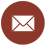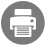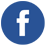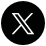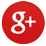Prof.
Michele Mossa
PhD
Professor of Hydraulics at the
Polytechnic University of Bari
POLYTECHNIC UNIVERSITY OF BARI
DICATECh
Department of Civil, Environmental, Land, Building Engineering and Chemistry
Via E. Orabona, 4 - 70125 Bari - ITALY

www.dicatech.poliba.it

LIC
Coastal Engineering Laboratory
Area Universitaria di Valenzano